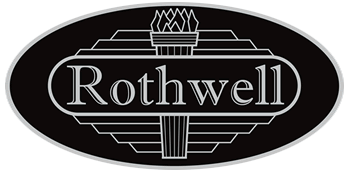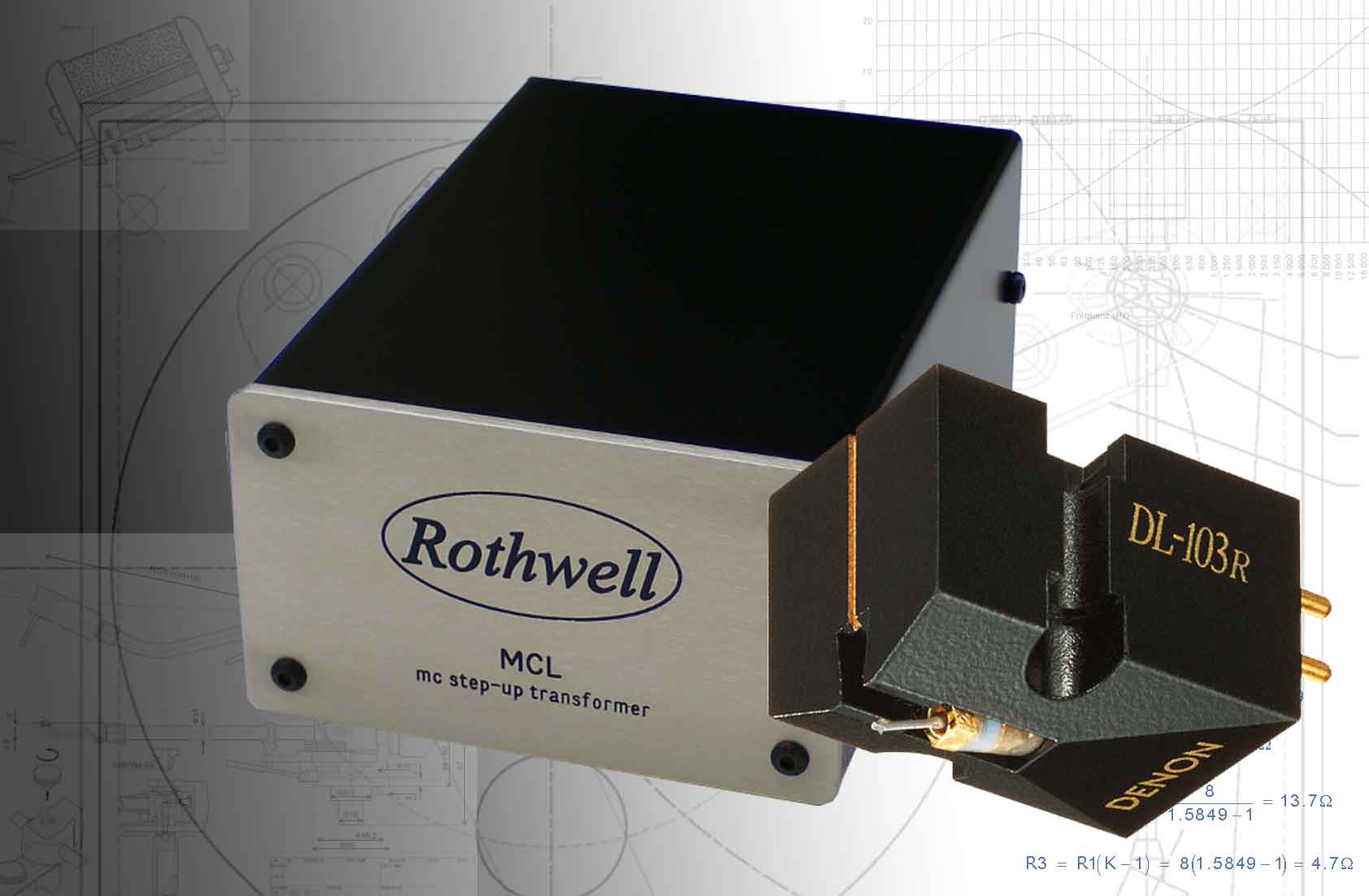step-up transformers for Denon cartridgesDenon moving coil cartriges are very popular and audiophiles looking to get the best performance from them often turn to step-up transformers in their quest for the ultimate quality - but some of the Denons aren't so easy to match with a transformer due to their higher than average source impedance and lower than average output voltage. So what is the correct transformer ratio? That depends to some extent on which particular cartridge you have, though the calculations show that there is a "one size fits all" solution for the low output moving coils with surprisingly little compromise. DL-304This cartridge has a source impedance of 40 ohms but a low output voltage of only 0.18mV. Attempting to achieve a signal voltage of 5mV into the following mm phonostage might suggest a transformer turns ratio of 1:28 would be a good idea, but such a ratio would result in the cartridge seeing a load impedance of only 60 ohms when the transformer is connected to a normal 47k mm phonostage input - too low to be ideal for a cartridge with a source impedance of 40 ohms. There would be a loss of signal of about 4.5dB at the cartridge/transformer interface and the resulting output voltage would be only 3mV, not 5mV. Still, 3mV is sufficient voltage for most mm phonostages to handle, though the signal-to-noise ratio would be less than if it had a higher signal to work with. So what if we tried a higher ratio? What about 1:40?Unfortunately, that doesn't really help. A higher ratio means the cartridge will see an even lower source impedance and even more signal will be lost at the cartridge/transformer interface. 1:40 gives the cartridge a load impedance of just 29 ohms to work with and the resulting output voltage will be 3mV - ie, no better than before. In fact, the performance is likely to be worse than with a 1:28 ratio because a higher turns ratio can only be achieved by either having more turns of wire on the transformer's secondary winding or by having fewer turns of wire on the primary winding. Putting more turns of wire on the secondary adds resistance and capacitance and results in degraded high frequency performance and often produces a resonant peak in the frequency response with its associated poor transient performance. Alternatively, fewer turns on the primary winding results in a lower primary inductance which degrades the low frequency response. Because of this it is good practice to keep the turns ratio as low as possible.So what is the optimum ratio for the DL-304? Well, if it was possible to make theoretically perfect transformers with infinite primary inductance, zero leakage inductance, zero capacitance and zero resistance, 1:35 would be optimum and yield a voltage step-up of 24.68dB. However, in the real world, transformer performance suffers as the ratio is increased and it is a good idea to use a low ratio rather than a high ratio wherever possible. If we are prepared to sacrifice just 1dB of voltage gain we only need a transformer ratio of 1:21, and the improved bandwidth is certainly worth the 1dB of signal loss. Transformer ratios of 1:20 are more common, and will give a voltage gain only 0.2dB less than 1:21.So, in practice a very good choice of ratio for a Denon DL-304 is 1:20 giving a voltage into the mm phonostage of 2.7mV. A ratio of 1:35 would give a voltage of3.08mV into the phonostage but at the cost of reduced bandwidth compared to a 1:20 transformer.Finally, there's one other approach we could take in our search for the optimum transformer turns ratio, and that's the recommended load impedance. Although aiming for a specific load impedance isn't normally an approach the author would recommend, it doesn't do any harm to look at the figures. Unfortunately, as is often the case, the manufacturer doesn't have a specific recommended load for the DL-304, only that it should be greater than 100 ohms. A turns ratio of 1:21 would give a load impedance of 106 ohms and a 1:20 ratio would give 117.5 ohms (assuming a standard 47k input impedance for the following phonostage). A 1:35 ratio would give us only 38 ohms, way below Denon's recommendation.Again, the maths appears to be steering us towards a ratio of 1:20 as the optimum for the DL-304, confirming the choice made by looking for a healthy voltage step-up.A suitable transformer from the Rothwell range would be the MCL. DL-103At 0.3mV this cartridge has a higher output voltage than the DL-304 but has the same source impedance of 40 ohms, therefore the same calculations apply when looking for the optimum turns ratio. 1:35 is the theoretical optimum for maximum voltage gain and gives 5.1mV into the phonostage. However, a transformer with a turns ratio of 1:20 will give an output voltage just 1.2dB lower at 4.5mV and will have better performance for the reasons outlined above.A suitable transformer from the Rothwell range would be the MCL. DL-103RThis cartridge is a revised version of the DL103 and has a slightly lower output voltage of 0.25mV, but has the advantage of a significantly lower source impedance of 14 ohms. The theoretical optimum turns ratio for maximum voltage transfer with this cartridge is 1:60. However, 1:60 is a huge ratio with serious practical compromises on performance. Even if it was possible to get acceptable performance from a 1:60 transformer there would still be another issue: output voltage. The output voltage from the transformer which would be fed into the phonostage would be 15mV - significantly more than a typical moving magnet cartridge - and would risk overload or severely curtailed headroom. Clearly, aiming for maximum voltage from the transformer has led to a poor choice for the turns ratio.So what would be a better ratio? A sensible criterion would be to aim for 5mV output from the transformer. This would give a good signal level into the following phonostage and give a good signal-to-noise ratio without the risk of overload, and the lower turns ratio required for 5mV would give better performance. The turns ratio required for a 5mV output would be 1:24. However, a 1:20 ratio would give still produce 4.5mV - only 1dB less than the 1:24 transformer. Anything in the range 1:20 to 1:24 would be a very good choice for the DL-103R.A suitable transformer from the Rothwell range would be the MCL. DL-301mkIIThis cartridge has a source impedance of 33 ohms and an output voltage of 0.4mV. The relevant calculations show that the optimum turns ratio for maximum output from the transformer is 1:40. However, 1:40 is again a high ratio with practical limitations on performance and would again result in a high signal voltage into the following phonostage of 7.5mV. What turns ratio would give us 5mV? 1:14 would get closest at 4.9mV.1:10 would give 3.7mV, only 2.6dB lower than the target figure. 1:20 would give 6.2mV, only 1.9dB above the target figure.Based on these figures anything in the range from 1:10 to 1:20 would be a good choice for the DL-301mkII.Suitable transformers from the Rothwell range would be the MC1, MCX and MCL. DL-110This is a high output moving coil and would normally be fed directly into an mm phonostage. Its source impedance is 160 ohms but its output voltage is only 1.6mV, which is lower than a typical mm cartridge. So, would it be possible to use a transformer to squeeze a bit more signal voltage out? The answer is “yes”, but it has to be the right transformer. What’s required is a low turns ratio of about 1:3. A 1:3 transformer would produce 4.7mV at its output, which would be a good figure and would produce a worthwhile improvement in signal-to-noise ratio from the phonostage.MC step-up transformers with such a low ratio are rare, but the MC1H is perfect for the job.However, most DL110 owners are happy to run the cartridge directly into an mm phonostage. Headamp alternativeInstead of using a step-up transformer it is possible to use a headamp instead. A headamp does essentially the same job as a step-up transformer but does it with an amplifier rather than transformers. The advantage is that the load impedance and gain can be set independently of each other and the bandwidth can be very wide, though transformers still have the edge for lowest possible noise.The Headspace is a low noise headamp with four gain settings and eight load impedance settings and can handle the full range of Denon cartridges.[Home] [Hi-Fi] [Attenuators] [Indus SE] [Rivers] [Hi-Fi dealers] [moving coil step-up transformer] [about Rothwell] [contact] [Rialto mm/mc phonostage] [mc step-up transformers explained] [mc step-up transformer] [valve phonostage] [SUT] [Simplex MM phonostage] [step-up transformers for Denon cartridges] [Signature One phonostage] [Headspace mc headamp] [SUT for HOMC] [Signature Two mc phonostage] [Custom step-up transformer] [Guitar] [shop]